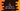# 3 different C programs to find the sum of first n even natural numbers## C program to find the sum of first n even natural numbers:

This post will show you how to find the sum of first n even natural numbers in C. The program will take the value of n as an input from the user and it will print the sum of first n even natural numbers.

A number is called an even number if it is divisible by 2. To find the sum of first n even numbers, we will have to run a loop that will run for infinite amount of time. We will also use another counter variable to keep the count of even numbers found. Once the count will be equal to n, it will exit. The sum of all even numbers can be stored in a separate sum variable.

I will show you different ways to find the sum of first n even numbers in this post.

### Algorithm:

The program will follow the below algorithm:

• Take the value of n from the user.
• Initialize a variable as 0 to hold the sum. Also, initialize another variable as 0 to hold the count.
• Run an infinite loop starting from 1. On each iteration of the loop, check if the current value is even or not. If yes, add it to the sum variable and increment the value of count by 1.
• If the value of count is equal to n exit from the loop.

### Method 1: C program with a for loop:

Below is the complete C program that uses the above algorithm:

``````#include <stdio.h>

int getEvenSum(int n)
{
int sum = 0, count = 0;

for (int i = 2;; i++)
{
if (i % 2 == 0)
{
sum += i;
count++;
}
if (count == n)
break;
}
return sum;
}

int main()
{
int n;

printf("Enter the value value of n: ");
scanf("%d", &n);

printf("Sum of first %d even numbers is: %d\n", n, getEvenSum(n));
}``````

Here,

• getEvenSum method is used to get the sum of first n even numbers.
• This method uses a for loop to run from 2. Inside the loop, it checks if the current value is an even number or not. If yes, it adds that value to a sum variable. This is initialized as 0.
• On every even number found, the value of count is incremented by 1. It is 0 at the start of the method. So, when it become equal to n, it exits from the loop.
• This method returns the value of sum.

If you run this program, it will print output as like below:

``````Enter the value value of n: 5
Sum of first 5 even numbers is: 30``````

### Method 2: C program with a while loop:

We can also use a while loop to write the same program. This program will work in a same way. The while loop will run until the value of count is less than or equal to n.

``````#include <stdio.h>

int getEvenSum(int n)
{
int sum = 0, count = 1, i = 2;

while (count <= n)
{
if (i % 2 == 0)
{
sum += i;
count++;
}

i += 1;
}
return sum;
}

int main()
{
int n;

printf("Enter the value value of n: ");
scanf("%d", &n);

printf("Sum of first %d even numbers is: %d\n", n, getEvenSum(n));
}``````
• getEvenSum method is used to get the sum of first n even numbers. It uses a while loop to find the required sum.
• The while loop will run until count ≤ n
• Inside the loop, we are adding the current value of i to the sum variable if it is even. Also, the value of count is incremented by 1.

It will print similar output.

### Method 3: By incrementing 2 on each iteration:

We can also reduce the total number or iteration by half. In the above examples, we are incrementing the value of i by 1 on each iteration and also checking if it is even or odd. Instead of this, we can increment the value of i by 2 on each iteration. If we start from 2, the loop will iterate over 2, 4, 6, 8, 10 etc. i.e. all even numbers.

``````#include <stdio.h>

int getEvenSum(int n)
{
int sum = 0, count = 0;

for (int i = 2;; i += 2)
{
sum += i;
count++;

if (count == n)
break;
}
return sum;
}

int main()
{
int n;

printf("Enter the value value of n: ");
scanf("%d", &n);

printf("Sum of first %d even numbers is: %d\n", n, getEvenSum(n));
}``````

This example is using a for loop. We don’t have to check if the current value is even or not. We can also write the same program using a while loop.

``````#include <stdio.h>

int getEvenSum(int n)
{
int sum = 0, count = 1, i = 2;

while (count <= n)
{
sum += i;
count++;

i += 2;
}
return sum;
}

int main()
{
int n;

printf("Enter the value value of n: ");
scanf("%d", &n);

printf("Sum of first %d even numbers is: %d\n", n, getEvenSum(n));
}``````

It will give similar output.